## Combine Data in Cells Using the CONCATENATE Operator or Functions

by Avantix Learning Team | Updated September 7, 2023

Applies to: Microsoft® Excel® 2013, 2016, 2019, 2021 and 365 (Windows)

You can combine the data from multiple cells into another cell using the CONCATENATE operator or CONCATENATE functions. CONCATENATE is often used to combine text in cells (like first name and last name) but you can also combine text with numbers, dates, functions, spaces, commas or dashes. If you have Excel 2019 or a later version, you can also use the CONCAT function. It is important to note that combining cells is different from merging cells.

Recommended article: How to Merge Cells in Excel (4 Ways)

Do you want to learn more about Excel? Check out our virtual classroom or in-person Excel courses >

In this article, we'll review 3 ways to combine cells using CONCATENATE:

If you want to combine dashes, commas, spaces or other text in an expression with CONCATENATE, you need to enter it in quotation marks or double quotes (such as " ").

If you want to enter formulas in an Excel table, you can use structured reference formulas which must refer to fields in square brackets []. You would also need to precede the field name with @ to refer to the current record.

### 1. Combine cells using the CONCATENATE operator (&)

You can use the CONCATENATE operator (&) to combine cells into one cell using a formula. Using the CONCATENATE operator, you can combine multiple cells and add other text or items in the expression.

In order to use the CONCATENATE operator, you will need to include it the formula each time you want to add something new to the expression.

To combine cells by entering a formula in Excel using the CONCATENATE operator:

1. Select the worksheet with the data you want to combine.
2. Click the cell where you want to insert the combined data.
3. Type an equal sign (=).
4. Type the cell reference for the first cell you want to combine or click it.
5. Type the CONCATENATE operator (&) by pressing Shift + 7 (at the top of the keyboard).
6. Type the cell reference for the cell you want to combine or click it.
7. Repeat for other cells or items you want to add. If you want to add text, enter it in quotation marks or double quotes.
8. Press Enter.

For example, if you wanted to combine the data from cells A2 and B2 (such as first name and last name), you could enter the following formula in C2:

=A2 & B2

If you wanted to add a space between the data, you would enter the following formula in C2:

=A2 &" " &B2

In the following example, we've entered a formula =A2 &" " &B2 in C2 and then copied the formula down to the cells below by dragging the Fill handle on the bottom right corner of the cell: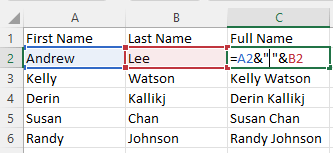In the following example, we've entered a formula in C2 in an Excel table and pressed Enter (Excel will populate the table column automatically):

=[@[First Name]] &" " &[@[Last Name]]

In the table example below, the structured reference formula using the CONCATENATE operator is entered in C2 and Excel color codes the references: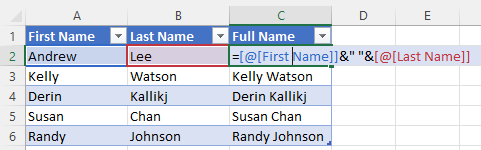If there are no spaces in the field names, you can enter the following formula in C2 in the table as follows:

=[@FirstName]& " " &[@LastName]

If you are creating structured reference formulas in Excel tables, it's easier if the field names do not include spaces.

### 2. Combine cells using the CONCATENATE function

You can also use the CONCATENATE function to combine cells into one cell using a formula. Using the CONCATENATE function, you can combine multiple cells and add other text or items in the expression.

With the CONCATENATE function, you simply include the items you want to combine in the arguments.

The Excel syntax for the CONCATENATE function is:

=CONCATENATE(text1, [text2], …)

The simple syntax for the CONCATENATE function is:

=CONCATENATE(item1, item2, item3, etc.)

Items could be text, spaces, commas, dashes, numbers, dates or other functions.

To combine cells by entering a formula in Excel using the CONCATENATE function:

1. Select the worksheet with the data you want to combine.
2. Click the cell where you want to insert the combined data.
3. Type an equal sign (=).
4. Type CONCATENATE and an open round bracket or parentheses (.
5. Enter the first cell or item you want to combine (such as A2).
6. Type a comma (,) to separate the arguments.
7. Enter the next cell or item you want to combine (such as " ").
8. Type a comma.
9. Repeat for other cells or items you want to combine. If you want to add text, enter it in quotation marks or double quotes.
10. Type a closed round bracket or parentheses ).
11. Press Enter.

For example, if you wanted to combine the data from cells A2 and B2 (such as first name and last name), you could enter the following formula in C2:

=CONCATENATE(A2, B2)

If you wanted to add a space between the data, you could enter the following formula in C2:

=CONCATENATE(A2, " ", B2)

If you wanted to add a comma between the data, you could enter the following formula in C2:

=CONCATENATE(B2, ", ", A2)

In the following example, we've entered a formula in C2 and then copied the formula down to the cells below by dragging the Fill handle on the bottom right corner of the cell:

=CONCATENATE(A2, ", ", B2)

In the example below, the formula using the CONCATENATE function is entered in C2 and Excel color codes the references: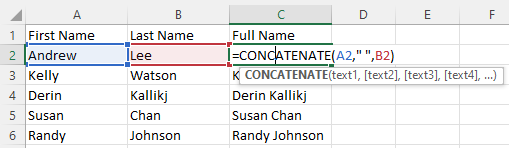In the following example, we've entered a formula in C2 in an Excel table and pressed Enter (Excel will populate the table column automatically):

=CONCATENATE([@[First Name]], " ", [@[Last Name]])

In the table example below, the structured reference formula using the CONCATENATE function is entered in C2 and Excel color codes the references: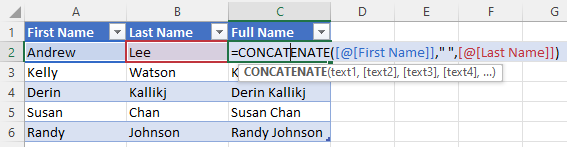In there are no spaces in the field names, you can enter the formula in C2 in the table as follows:

=CONCATENATE([@FirstName], " " , [@LastName])

### 3. Combine cells using the CONCAT function

If you have Excel 2019 or a later version, you can also use the CONCAT function to combine cells. This function allows you to quickly combine two or more text strings together including a range of cells (such product codes).

You can use the CONCAT function to combine cells in another cell using a formula. Using the CONCAT function, you can combine multiple cells and add other text or items in the expression.

The Excel syntax for the CONCAT function is:

=CONCAT(text1, [text2, … text_n], …)

The simple syntax for the CONCAT function is:

=CONCAT(item1, item2, item3, etc.) or =CONCAT(item1:itemN)

Items could be text, spaces, commas, dashes, numbers, dates or other functions.

In many cases, the CONCAT function behaves the same way as the CONCATENATE function. However, you can use ranges of cells with the CONCAT function.

For example:

=CONCAT(A2:C2)

To combine cells by entering a formula in Excel using the CONCAT function:

1. Select the worksheet with the data you want to combine.
2. Click the cell where you want to insert the combined data.
3. Type an equal sign (=).
4. Type CONCAT and an open round bracket or parentheses (.
5. Enter the first cell or item you want to combine (such as A2). Alternatively, you can enter a range of cells (such as A2:C2).
6. Type a comma (,) to separate the arguments.
7. Enter the next cell or item you want to combine (such as " ").
8. Type a comma.
9. Repeat for other cells or items you want to combine. If you want to add text, enter it in quotation marks or double quotes.
10. Type a closed round bracket or parentheses ).
11. Press Enter.

For example, if you wanted to combine the data from cells A2 to C2 (such as color, styles and category), you could enter the following formula in D2:

=CONCAT(A2:C2)

If you wanted to add a dash between the data, you could enter the following formula in D2:

=CONCAT(A2, "-", B2, "-", C2)

In the following example, we've entered a formula =CONCAT(A2, " ", B2) in C2: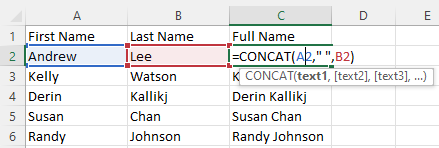In the following example, we've entered a formula =CONCAT(A2:C2) in D2: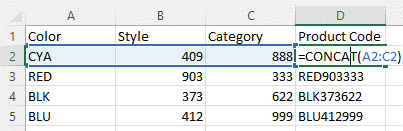In the following example, we've entered a formula in C2 in an Excel table pressed Enter (Excel will populate the table column automatically):

=CONCAT([@[First Name]], " ", [@[Last Name]])

In the table example below, the structured reference formula using the CONCAT function is entered in C2 and Excel color codes the references: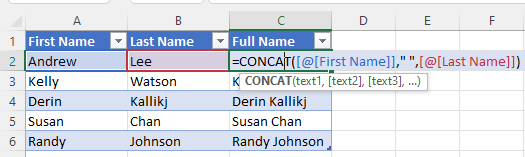If there are no spaces in the field names, you can enter the formula in C2 in the table as follows:

=CONCAT([@FirstName],  " ", [@LastName])

You can combine the CONCATENATE operator and functions with other functions such as TRIM and UPPER.

There are also others ways to combine data including using Flash Fill and the TEXTJOIN function.

#### More resources

How to Remove Duplicates in Excel (3 Easy Ways)

How to Combine First Name and Last Name in Excel (5 Ways)

How to Insert Multiple Rows in Excel (4 Fast Ways with Shortcuts)

Use Conditional Formatting in Excel to Highlight Dates Before Today (3 Ways)

How to Replace Blank Cells in Excel with Zeros (0), Dashes (-) or Other Values

#### Related courses

Microsoft Excel: Data Analysis with Functions, Dashboards and What-If Analysis Tools

Microsoft Excel: Introduction to Power Query to Get and Transform Data

Microsoft Excel: New and Essential Features and Functions in Excel 365

Microsoft Excel: Introduction to Visual Basic for Applications (VBA)

VIEW MORE COURSES >

Our instructor-led courses are delivered in virtual classroom format or at our downtown Toronto location at 18 King Street East, Suite 1400, Toronto, Ontario, Canada (some in-person classroom courses may also be delivered at an alternate downtown Toronto location). Contact us at info@avantixlearning.ca if you'd like to arrange custom instructor-led virtual classroom or onsite training on a date that's convenient for you.

## How to Use Flash Fill in Excel (4 Ways with Shortcuts)

You can use Flash Fill in Excel to extract, combine, clean or format data quickly without using formulas. In order to use Flash Fill, Excel must be able to understand a pattern in a column to the left of the column where you want to fill the data so the source data should be entered in a consistent way. You can use Flash Fill by clicking a button, using shortcuts or by using the Fill handle. You run Flash Fill by entering an example of the data you want to fill using a "by example" logic.

Microsoft, the Microsoft logo, Microsoft Office and related Microsoft applications and logos are registered trademarks of Microsoft Corporation in Canada, US and other countries. All other trademarks are the property of the registered owners.

Avantix Learning |18 King Street East, Suite 1400, Toronto, Ontario, Canada M5C 1C4 | Contact us at info@avantixlearning.ca

Summary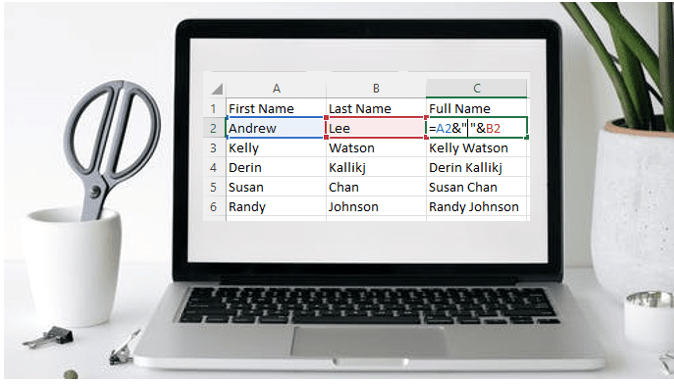Article Name
How to Combine Cells in Excel Using Concatenate (3 Ways)
Description
You can combine the data from multiple cells into another cell using the CONCATENATE operator or CONCATENATE functions. CONCATENATE is often used to combine text in cells (like first name and last name) but you can also combine text with numbers, dates, functions, spaces, commas or dashes. If you have Excel 2019 or a later version, you can also use the CONCAT function. It is important to note that combining cells is different from merging cells.
Author
Publisher Name
Avantix Learning